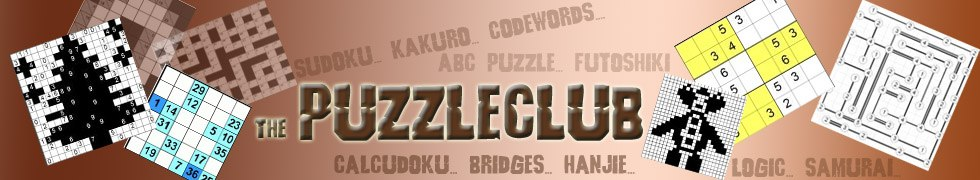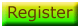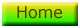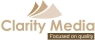# Number Hexagon Rules

In the number hexagon, there are some triangles that have numbers in them and some that don't.

The aim of the puzzle is simply to fill each triangle with a number such that the sum total of the triangles that compose each hexagon is that given in the instructions on the first page of each puzzle.

You can't repeat numbers within a hexagon and this is the key to working out how to solve the puzzles, together with knowledge of addition and which numbers can be used to make which other number!©   |     |